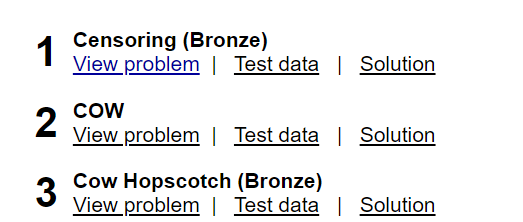# Censoring

Here is the problem statement: USACO

Guys I just need to confirm if I understand the question right. Here are my thoughts:
String S and T, so I use while loop until there aren’t any T in S as the statement said. “tmp” is the string S after it has been removed all of T’s appearances, “cnt” count the appearances of string T in S.
I have tried so many cases but only got test 1,2,3,6 right. Is there any special case I missed?
I’d appreciated if someone helped me :))))
Here is my code:

``````#include <bits/stdc++.h>
using namespace std;

string s,t;

int main()
{
freopen("censor.in","r",stdin);
freopen("censor.out","w",stdout);

cin>>s>>t;

while(1){
string tmp;
int j=0,cnt=0,i=0;

while(i<s.length()){
if(s[i]!=t[j]){
tmp+=s[i];
i++;
}
else{
string m;
while(i<s.length() && s[i]==t[j] && j<t.length()){
m+=s[i];
i++;
j++;
}
if(j<t.length()){
tmp+=m;
}
else cnt++;
j=0;

}
}

s=tmp;

if(cnt==0) break;
}

cout<<s;

return 0;
}

``````